# Select Rows & Columns by Name or Index in Pandas DataFrame using [ ], loc & iloc

Indexing in Pandas means selecting rows and columns of data from a Dataframe. It can be selecting all the rows and the particular number of columns, a particular number of rows, and all the columns or a particular number of rows and columns each. Indexing is also known as Subset selection.
Let’s create a simple dataframe with a list of tuples, say column names are: ‘Name’, ‘Age’, ‘City’ and ‘Salary’.

 `# import pandas ` `import` `pandas as pd ` ` `  `# List of Tuples ` `employees ``=` `[(``'Stuti'``, ``28``, ``'Varanasi'``, ``20000``), ` `            ``(``'Saumya'``, ``32``, ``'Delhi'``, ``25000``), ` `            ``(``'Aaditya'``, ``25``, ``'Mumbai'``, ``40000``), ` `            ``(``'Saumya'``, ``32``, ``'Delhi'``, ``35000``), ` `            ``(``'Saumya'``, ``32``, ``'Delhi'``, ``30000``), ` `            ``(``'Saumya'``, ``32``, ``'Mumbai'``, ``20000``), ` `            ``(``'Aaditya'``, ``40``, ``'Dehradun'``, ``24000``), ` `            ``(``'Seema'``, ``32``, ``'Delhi'``, ``70000``) ` `            ``] ` ` `  `# Create a DataFrame object from list  ` `df ``=` `pd.DataFrame(employees,  ` `                ``columns ``=``[``'Name'``, ``'Age'``,  ` `                         ``'City'``, ``'Salary'``]) ` `# Show the dataframe ` `df `

Output: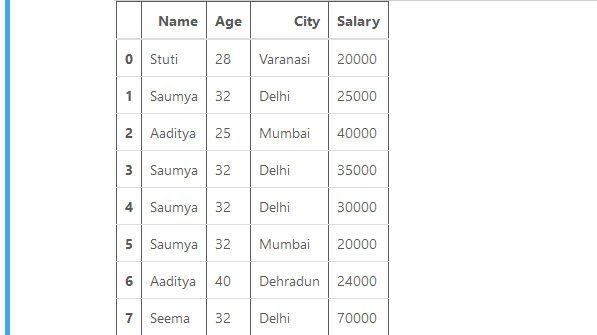Method 1: using Dataframe.[ ].
[ ] is used to select a column by mentioning the respective column name.

Example 1 : to select single column.
Code:

 `# import pandas ` `import` `pandas as pd ` ` `  `# List of Tuples ` `employees ``=` `[(``'Stuti'``, ``28``, ``'Varanasi'``, ``20000``), ` `            ``(``'Saumya'``, ``32``, ``'Delhi'``, ``25000``), ` `            ``(``'Aaditya'``, ``25``, ``'Mumbai'``, ``40000``), ` `            ``(``'Saumya'``, ``32``, ``'Delhi'``, ``35000``), ` `            ``(``'Saumya'``, ``32``, ``'Delhi'``, ``30000``), ` `            ``(``'Saumya'``, ``32``, ``'Mumbai'``, ``20000``), ` `            ``(``'Aaditya'``, ``40``, ``'Dehradun'``, ``24000``), ` `            ``(``'Seema'``, ``32``, ``'Delhi'``, ``70000``) ` `            ``] ` ` `  `# Create a DataFrame object from list  ` `df ``=` `pd.DataFrame(employees,  ` `                ``columns ``=``[``'Name'``, ``'Age'``,  ` `                         ``'City'``, ``'Salary'``]) ` ` `  `# Using the operator []  ` `# to select a column ` `result ``=` `df[``"City"``] ` ` `  `# Show the dataframe ` `result `

Output: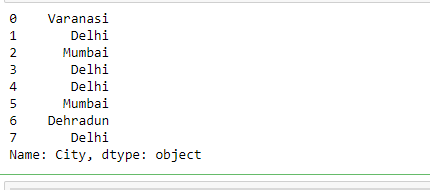Example 2: to select multiple columns.
Code:

 `# import pandas ` `import` `pandas as pd ` ` `  `# List of Tuples ` `employees ``=` `[(``'Stuti'``, ``28``, ``'Varanasi'``, ``20000``), ` `            ``(``'Saumya'``, ``32``, ``'Delhi'``, ``25000``), ` `            ``(``'Aaditya'``, ``25``, ``'Mumbai'``, ``40000``), ` `            ``(``'Saumya'``, ``32``, ``'Delhi'``, ``35000``), ` `            ``(``'Saumya'``, ``32``, ``'Delhi'``, ``30000``), ` `            ``(``'Saumya'``, ``32``, ``'Mumbai'``, ``20000``), ` `            ``(``'Aaditya'``, ``40``, ``'Dehradun'``, ``24000``), ` `            ``(``'Seema'``, ``32``, ``'Delhi'``, ``70000``) ` `            ``] ` ` `  `# Create a DataFrame object from list  ` `df ``=` `pd.DataFrame(employees,  ` `                ``columns ``=``[``'Name'``, ``'Age'``, ` `                        ``'City'``, ``'Salary'``]) ` ` `  `# Using the operator [] to  ` `# select multiple columns ` `result ``=` `df[[``"Name"``, ``"Age"``, ``"Salary"``]] ` ` `  `# Show the dataframe ` `result `

Output: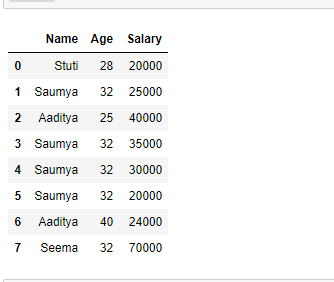Method 2: Using Dataframe.loc[ ].
.loc[] the function selects the data by labels of rows or columns. It can select a subset of rows and columns. There are many ways to use this function.
Example 1: To select single row.
Code:

 `# import pandas ` `import` `pandas as pd ` ` `  `# List of Tuples ` `employees ``=` `[(``'Stuti'``, ``28``, ``'Varanasi'``, ``20000``), ` `            ``(``'Saumya'``, ``32``, ``'Delhi'``, ``25000``), ` `            ``(``'Aaditya'``, ``25``, ``'Mumbai'``, ``40000``), ` `            ``(``'Saumya'``, ``32``, ``'Delhi'``, ``35000``), ` `            ``(``'Saumya'``, ``32``, ``'Delhi'``, ``30000``), ` `            ``(``'Saumya'``, ``32``, ``'Mumbai'``, ``20000``), ` `            ``(``'Aaditya'``, ``40``, ``'Dehradun'``, ``24000``), ` `            ``(``'Seema'``, ``32``, ``'Delhi'``, ``70000``) ` `            ``] ` ` `  `# Create a DataFrame object from list  ` `df ``=` `pd.DataFrame(employees, ` `                 ``columns ``=``[``'Name'``, ``'Age'``, ` `                  ``'City'``, ``'Salary'``]) ` ` `  `# Set 'Name' column as index  ` `# on a Dataframe ` `df.set_index(``"Name"``, inplace ``=` `True``) ` ` `  `# Using the operator .loc[] ` `# to select single row ` `result ``=` `df.loc[``"Stuti"``] ` ` `  `# Show the dataframe ` `result `

Output: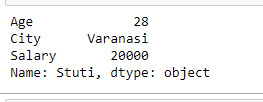Example 2: To select multiple rows.
Code:

 `# import pandas ` `import` `pandas as pd ` ` `  `# List of Tuples ` `employees ``=` `[(``'Stuti'``, ``28``, ``'Varanasi'``, ``20000``), ` `            ``(``'Saumya'``, ``32``, ``'Delhi'``, ``25000``), ` `            ``(``'Aaditya'``, ``25``, ``'Mumbai'``, ``40000``), ` `            ``(``'Saumya'``, ``32``, ``'Delhi'``, ``35000``), ` `            ``(``'Saumya'``, ``32``, ``'Delhi'``, ``30000``), ` `            ``(``'Saumya'``, ``32``, ``'Mumbai'``, ``20000``), ` `            ``(``'Aaditya'``, ``40``, ``'Dehradun'``, ``24000``), ` `            ``(``'Seema'``, ``32``, ``'Delhi'``, ``70000``) ` `            ``] ` ` `  `# Create a DataFrame object from list  ` `df ``=` `pd.DataFrame(employees,  ` `                  ``columns ``=``[``'Name'``, ``'Age'``, ` `                   ``'City'``, ``'Salary'``]) ` ` `  `# Set index on a Dataframe ` `df.set_index(``"Name"``,  ` `              ``inplace ``=` `True``) ` ` `  `# Using the operator .loc[] ` `# to select multiple rows ` `result ``=` `df.loc[[``"Stuti"``, ``"Seema"``]] ` ` `  `# Show the dataframe ` `result `

Output: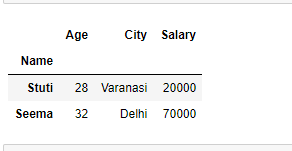Example 3: To select multiple rows and particular columns.

`Syntax:  Dataframe.loc[["row1", "row2"...], ["column1", "column2", "column3"...]]`

Code:

 `# import pandas ` `import` `pandas as pd ` ` `  `# List of Tuples ` `employees ``=` `[(``'Stuti'``, ``28``, ``'Varanasi'``, ``20000``), ` `            ``(``'Saumya'``, ``32``, ``'Delhi'``, ``25000``), ` `            ``(``'Aaditya'``, ``25``, ``'Mumbai'``, ``40000``), ` `            ``(``'Saumya'``, ``32``, ``'Delhi'``, ``35000``), ` `            ``(``'Saumya'``, ``32``, ``'Delhi'``, ``30000``), ` `            ``(``'Saumya'``, ``32``, ``'Mumbai'``, ``20000``), ` `            ``(``'Aaditya'``, ``40``, ``'Dehradun'``, ``24000``), ` `            ``(``'Seema'``, ``32``, ``'Delhi'``, ``70000``) ` `            ``] ` ` `  `# Create a DataFrame object from list  ` `df ``=` `pd.DataFrame(employees,  ` `                 ``columns ``=``[``'Name'``, ``'Age'``, ` `                  ``'City'``, ``'Salary'``]) ` ` `  `# Set 'Name' column as index  ` `# on a Dataframe ` `df.set_index(``"Name"``, inplace ``=` `True``) ` ` `  `# Using the operator .loc[] to  ` `# select multiple rows with some ` `# particular columns ` `result ``=` `df.loc[[``"Stuti"``, ``"Seema"``], ` `               ``[``"City"``, ``"Salary"``]] ` ` `  `# Show the dataframe ` `result `

Output: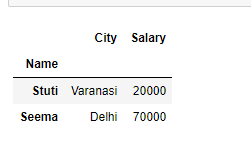Example 4: To select all the rows with some particular columns. We use single colon [ : ] to select all rows and list of columns which we want to select as given below :

`Syntax: Dataframe.loc[[:, ["column1", "column2", "column3"]]`

Code:

 `# import pandas ` `import` `pandas as pd ` ` `  `# List of Tuples ` `employees ``=` `[(``'Stuti'``, ``28``, ``'Varanasi'``, ``20000``), ` `            ``(``'Saumya'``, ``32``, ``'Delhi'``, ``25000``), ` `            ``(``'Aaditya'``, ``25``, ``'Mumbai'``, ``40000``), ` `            ``(``'Saumya'``, ``32``, ``'Delhi'``, ``35000``), ` `            ``(``'Saumya'``, ``32``, ``'Delhi'``, ``30000``), ` `            ``(``'Saumya'``, ``32``, ``'Mumbai'``, ``20000``), ` `            ``(``'Aaditya'``, ``40``, ``'Dehradun'``, ``24000``), ` `            ``(``'Seema'``, ``32``, ``'Delhi'``, ``70000``) ` `            ``] ` ` `  `# Creating a DataFrame object from list  ` `df ``=` `pd.DataFrame(employees, ` `                  ``columns ``=``[``'Name'``, ``'Age'``,  ` `                  ``'City'``, ``'Salary'``]) ` ` `  `# Set 'Name' column as index  ` `# on a Dataframe ` `df.set_index(``"Name"``, inplace ``=` `True``) ` ` `  `# Using the operator .loc[] to ` `# select all the rows with  ` `# some particular columns ` `result ``=` `df.loc[:, [``"City"``, ``"Salary"``]] ` ` `  `# Show the dataframe ` `result `

Output: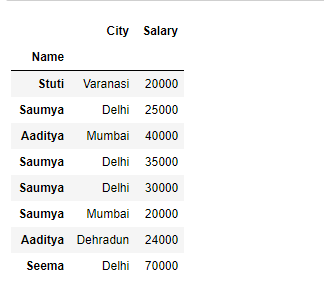Method 3: Using Dataframe.iloc[ ].
iloc[ ] is used for selection based on position. It is similar to loc[] indexer but it takes only integer values to make selections.
Example 1 : to select a single row.
Code:

 `# import pandas ` `import` `pandas as pd ` ` `  `# List of Tuples ` `employees ``=` `[(``'Stuti'``, ``28``, ``'Varanasi'``, ``20000``), ` `            ``(``'Saumya'``, ``32``, ``'Delhi'``, ``25000``), ` `            ``(``'Aaditya'``, ``25``, ``'Mumbai'``, ``40000``), ` `            ``(``'Saumya'``, ``32``, ``'Delhi'``, ``35000``), ` `            ``(``'Saumya'``, ``32``, ``'Delhi'``, ``30000``), ` `            ``(``'Saumya'``, ``32``, ``'Mumbai'``, ``20000``), ` `            ``(``'Aaditya'``, ``40``, ``'Dehradun'``, ``24000``), ` `            ``(``'Seema'``, ``32``, ``'Delhi'``, ``70000``) ` `            ``] ` ` `  `# Create a DataFrame object from list  ` `df ``=` `pd.DataFrame(employees,  ` `                  ``columns ``=``[``'Name'``, ``'Age'``, ` `                   ``'City'``, ``'Salary'``]) ` ` `  `# Using the operator .iloc[] ` `# to select single row ` `result ``=` `df.iloc[``2``] ` ` `  `# Show the dataframe ` `result `

Output: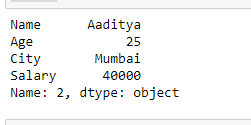Example 2: to select multiple rows.
Code:

 `# import pandas ` `import` `pandas as pd ` ` `  `# List of Tuples ` `employees ``=` `[(``'Stuti'``, ``28``, ``'Varanasi'``, ``20000``), ` `            ``(``'Saumya'``, ``32``, ``'Delhi'``, ``25000``), ` `            ``(``'Aaditya'``, ``25``, ``'Mumbai'``, ``40000``), ` `            ``(``'Saumya'``, ``32``, ``'Delhi'``, ``35000``), ` `            ``(``'Saumya'``, ``32``, ``'Delhi'``, ``30000``), ` `            ``(``'Saumya'``, ``32``, ``'Mumbai'``, ``20000``), ` `            ``(``'Aaditya'``, ``40``, ``'Dehradun'``, ``24000``), ` `            ``(``'Seema'``, ``32``, ``'Delhi'``, ``70000``) ` `            ``] ` ` `  `# Create a DataFrame object from list  ` `df ``=` `pd.DataFrame(employees,  ` `                ``columns ``=``[``'Name'``, ``'Age'``, ` `                ``'City'``, ``'Salary'``]) ` ` `  `# Using the operator .iloc[]  ` `# to select multiple rows ` `result ``=` `df.iloc[[``2``, ``3``, ``5``]] ` ` `  `# Show the dataframe ` `result `

Output: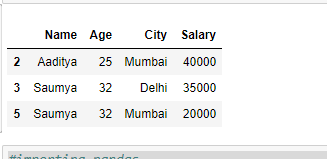Example 3: to select multiple rows with some particular columns.
Code:

 `# import pandas ` `import` `pandas as pd ` ` `  `# List of Tuples ` `employees ``=` `[(``'Stuti'``, ``28``, ``'Varanasi'``, ``20000``), ` `            ``(``'Saumya'``, ``32``, ``'Delhi'``, ``25000``), ` `            ``(``'Aaditya'``, ``25``, ``'Mumbai'``, ``40000``), ` `            ``(``'Saumya'``, ``32``, ``'Delhi'``, ``35000``), ` `            ``(``'Saumya'``, ``32``, ``'Delhi'``, ``30000``), ` `            ``(``'Saumya'``, ``32``, ``'Mumbai'``, ``20000``), ` `            ``(``'Aaditya'``, ``40``, ``'Dehradun'``, ``24000``), ` `            ``(``'Seema'``, ``32``, ``'Delhi'``, ``70000``) ` `            ``] ` ` `  `# Creating a DataFrame object from list  ` `df ``=` `pd.DataFrame(employees, ` `                  ``columns ``=``[``'Name'``, ``'Age'``, ` `                  ``'City'``, ``'Salary'``]) ` ` `  `# Using the operator .iloc[]  ` `# to select multiple rows with ` `# some particular columns ` `result ``=` `df.iloc[[``2``, ``3``, ``5``], ` `                  ``[``0``, ``1``]] ` ` `  `# Show the dataframe ` `result `

Output: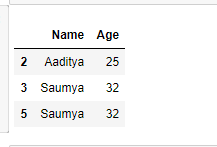Example 4: to select all the rows with some particular columns.
Code:

 `# import pandas ` `import` `pandas as pd ` ` `  `# List of Tuples ` `employees ``=` `[(``'Stuti'``, ``28``, ``'Varanasi'``, ``20000``), ` `            ``(``'Saumya'``, ``32``, ``'Delhi'``, ``25000``), ` `            ``(``'Aaditya'``, ``25``, ``'Mumbai'``, ``40000``), ` `            ``(``'Saumya'``, ``32``, ``'Delhi'``, ``35000``), ` `            ``(``'Saumya'``, ``32``, ``'Delhi'``, ``30000``), ` `            ``(``'Saumya'``, ``32``, ``'Mumbai'``, ``20000``), ` `            ``(``'Aaditya'``, ``40``, ``'Dehradun'``, ``24000``), ` `            ``(``'Seema'``, ``32``, ``'Delhi'``, ``70000``) ` `            ``] ` ` `  `# Create a DataFrame object from list  ` `df ``=` `pd.DataFrame(employees,  ` `                ``columns ``=``[``'Name'``, ``'Age'``,  ` `               ``'City'``, ``'Salary'``]) ` ` `  `# Using the operator .iloc[] ` `# to select all the rows with ` `# some particular columns ` `result ``=` `df.iloc[:, [``0``, ``1``]] ` ` `  `# Show the dataframe ` `result `

Output: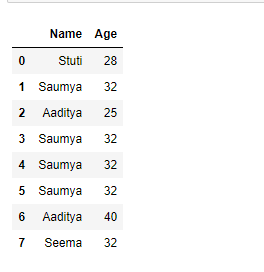Attention geek! Strengthen your foundations with the Python Programming Foundation Course and learn the basics.

To begin with, your interview preparations Enhance your Data Structures concepts with the Python DS Course.

My Personal Notes arrow_drop_upCheck out this Author's contributed articles.

If you like GeeksforGeeks and would like to contribute, you can also write an article using contribute.geeksforgeeks.org or mail your article to contribute@geeksforgeeks.org. See your article appearing on the GeeksforGeeks main page and help other Geeks.

Please Improve this article if you find anything incorrect by clicking on the "Improve Article" button below.

Article Tags :

Be the First to upvote.

Please write to us at contribute@geeksforgeeks.org to report any issue with the above content.